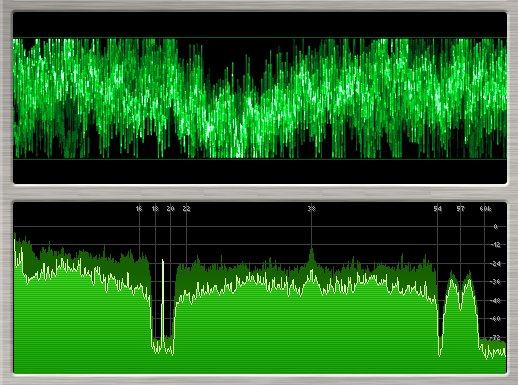•• The Difference between Bit Rate and Baud Rate

The difference between Bit and Baud rate is complicated and intertwining. Both are dependent and inter-related. But the simplest explanation is that a Bit Rate is how many data bits are transmitted per second. A baud Rate is the number of times per second a signal in a communications channel changes.

Bit rates measure the number of data bits (that is 0’s and 1’s) transmitted in one second in a communication channel. A figure of 2400 bits per second means 2400 zeros or ones can be transmitted in one second, hence the abbreviation “bps.” Individual characters (for example letters or numbers) that are also referred to as bytes are composed of several bits.

A baud rate is the number of times a signal in a communications channel changes state or varies. For example, a 2400 baud rate means that the channel can change states up to 2400 times per second. The term “change state” means that it can change from 0 to 1 or from 1 to 0 up to X (in this case, 2400) times per second. It also refers to the actual state of the connection, such as voltage, frequency, or phase level).The main difference between the two is that one change of state can transmit one bit, or slightly more or less than one bit, that depends on the modulation technique used. So the bit rate (bps) and baud rate (baud per second) have this connection:

bps = baud per second x the number of bit per baud

The modulation technique determines the number of bit per baud. Here are two examples:

When FSK (Frequency Shift Keying, a transmission technique) is used, each baud transmits one bit. Only one change in state is required to send a bit. Thus, the modem’s bps rate is equal to the baud rate. When a baud rate of 2400 is used, a modulation technique called phase modulation that transmits four bits per baud is used. So:

2400 baud x 4 bits per baud = 9600 bps

Such modems are capable of 9600 bps operation.